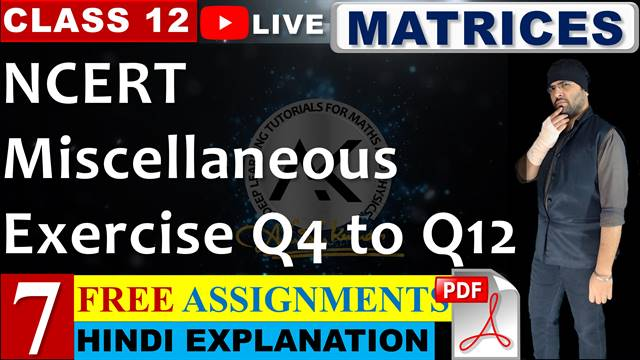# Matrices Class 12 Maths Chapter 3 Miscellaneous Exercise## Topics/Questions discussed in this video:

00:00:58 NCERT Solutions Miscellaneous Exercise Question 4
If A and B are symmetric matrices, prove that AB – BA is a skew symmetric matrix.

00:05:18 NCERT Solutions Miscellaneous Exercise Question 5
Show that the matrix B′AB is symmetric or skew symmetric according as A is symmetric or skew symmetric.

00:11:28 NCERT Solutions Miscellaneous Exercise Question 6 Find the values of x, y, z if the matrix A\, = \, {\begin{bmatrix} 0&{2y}&z\\ x&y&{ - z}\\ x&{ - y}&z \end{bmatrix}} satisfy the equation {\rm{A'A = I}}.

00:16:28 NCERT Solutions Miscellaneous Exercise Question 7 For what values of x : {\begin{bmatrix} 1&2&1 \end{bmatrix}} {\begin{bmatrix} 1&2&0\\ 2&0&1\\ 1&0&2 \end{bmatrix}} {\begin{bmatrix} 0\\ 2\\ x \end{bmatrix}} = {\rm{O}}?

00:18:48 NCERT Solutions Miscellaneous Exercise Question 8 If A = {\begin{bmatrix} 3&1\\ { - 1}&2 \end{bmatrix}} , show that {A^2} - 5A + 7I = O.

00:20:58 NCERT Solutions Miscellaneous Exercise Question 9 Find x, if {\begin{bmatrix} x&{ - 5}&{ - 1} \end{bmatrix}} {\begin{bmatrix} 1&0&2\\ 0&2&1\\ 2&0&3 \end{bmatrix}} {\begin{bmatrix} x\\ 4\\ 1 \end{bmatrix}} = {\rm{O}}

00:23:28 NCERT Solutions Miscellaneous Exercise Question 10 A manufacturer produces three products x, y, z which he sells in two markets. Annual sales are indicated below:
\begin{matrix} {\rm{Market}} & & & {\rm{Products}}\\ \,\,\,\,\,\,\,\begin{bmatrix} {\rm{I}}\\ {{\rm{II}}} \end{bmatrix} & & & \begin{bmatrix} {10000}&{2000}&{18000}\\ {6000}&{20000}&{8000} \end{bmatrix} \end{matrix}
(a) If unit sale prices of x, y and z are ₹ 2.50, ₹ 1.50 and ₹ 1.00, respectively, find the total revenue in each market with the help of matrix algebra.
(b) If the unit costs of the above three commodities are ₹ 2.00, ₹ 1.00 and 50 paise respectively. Find the gross profit.

00:35:28 NCERT Solutions Miscellaneous Exercise Question 11 Find the matrix X so that {\rm{X}} {\begin{bmatrix} 1&2&3\\ 4&5&6 \end{bmatrix}} = {\begin{bmatrix} { - 7}&{ - 8}&{ - 9}\\ 2&4&6 \end{bmatrix}}

Choose the correct answer in the following questions:

00:42:18 NCERT Solutions Miscellaneous Exercise Question 15 If A is square matrix such that {A^2} = A, then {(I + A)^3} - 7Ais equal to
(A) A
(B) I – A
(C) I
(D) 3A

00:45:08 NCERT Solutions Miscellaneous Exercise Question 14 If the matrix A is both symmetric and skew symmetric, then
(A) A is a diagonal matrix
(B) A is a zero matrix
(C) A is a square matrix
(D) None of these

00:46:48 NCERT Solutions Miscellaneous Exercise Question 13 If A = {\begin{bmatrix} \alpha &\beta \\ \gamma &{ - \alpha } \end{bmatrix}} is such that {{\rm{A}}^{\rm{2}}}{\rm{ = I}}, then
(A) 1 + {\alpha ^2} + \beta \gamma = 0
(B) 1 - {\alpha ^2} + \beta \gamma = 0
(C) 1 - {\alpha ^2} - \beta \gamma = 0
(D) 1 + {\alpha ^2} - \beta \gamma = 0

00:49:38 NCERT Solutions Miscellaneous Exercise Question 12 If A and B are square matrices of the same order such that AB = BA, then prove by induction that A{B^n} = {B^n}A. Further, prove that {(AB)^n} = {A^n}{B^n} for all n ∈ N.